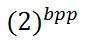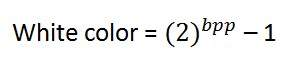# Concept of Bits Per Pixel

Bpp or bits per pixel denotes the number of bits per pixel. The number of different colors in an image is depends on the depth of color or bits per pixel.

## Bits in mathematics:

Its just like playing with binary bits.

How many numbers can be represented by one bit.

0

1

How many two bits combinations can be made.

00

01

10

11

If we devise a formula for the calculation of total number of combinations that can be made from bit, it would be like this.Where bpp denotes bits per pixel. Put 1 in the formula you get 2, put 2 in the formula, you get 4. It grows exponentially.

## Number of different colors:

Now as we said it in the beginning, that the number of different colors depend on the number of bits per pixel.

The table for some of the bits and their color is given below.

Bits per pixel Number of colors
1 bpp 2 colors
2 bpp 4 colors
3 bpp 8 colors
4 bpp 16 colors
5 bpp 32 colors
6 bpp 64 colors
7 bpp 128 colors
8 bpp 256 colors
10 bpp 1024 colors
16 bpp 65536 colors
24 bpp 16777216 colors (16.7 million colors)
32 bpp 4294967296 colors (4294 million colors)

This table shows different bits per pixel and the amount of color they contain.

You can easily notice the pattern of the exponentional growth. The famous gray scale image is of 8 bpp , means it has 256 different colors in it or 256 shades.Color images are usually of the 24 bpp format, or 16 bpp.

We will see more about other color formats and image types in the tutorial of image types.

### Color values:

We have previously seen in the tutorial of concept of pixel, that 0 pixel value denotes black color.

### Black color:

Remember, 0 pixel value always denotes black color. But there is no fixed value that denotes white color.

### White color:

The value that denotes white color can be calculated as :In case of 1 bpp, 0 denotes black, and 1 denotes white.

In case 8 bpp, 0 denotes black, and 255 denotes white.

### Gray color:

When you calculate the black and white color value, then you can calculate the pixel value of gray color.

Gray color is actually the mid point of black and white. That said,

In case of 8bpp, the pixel value that denotes gray color is 127 or 128bpp (if you count from 1, not from 0).

## Image storage requirements

After the discussion of bits per pixel, now we have every thing that we need to calculate a size of an image.

### Image size

The size of an image depends upon three things.

• Number of rows
• Number of columns
• Number of bits per pixel

The formula for calculating the size is given below.

Size of an image = rows * cols * bpp

It means that if you have an image, lets say this one:Assuming it has 1024 rows and it has 1024 columns. And since it is a gray scale image, it has 256 different shades of gray or it has bits per pixel. Then putting these values in the formula, we get

Size of an image = rows * cols * bpp

= 1024 * 1024 * 8

= 8388608 bits.

But since its not a standard answer that we recognize, so will convert it into our format.

Converting it into bytes = 8388608 / 8 = 1048576 bytes.

Converting into kilo bytes = 1048576 / 1024 = 1024kb.

Converting into Mega bytes = 1024 / 1024 = 1 Mb.

Thats how an image size is calculated and it is stored. Now in the formula, if you are given the size of image and the bits per pixel, you can also calculate the rows and columns of the image, provided the image is square(same rows and same column).